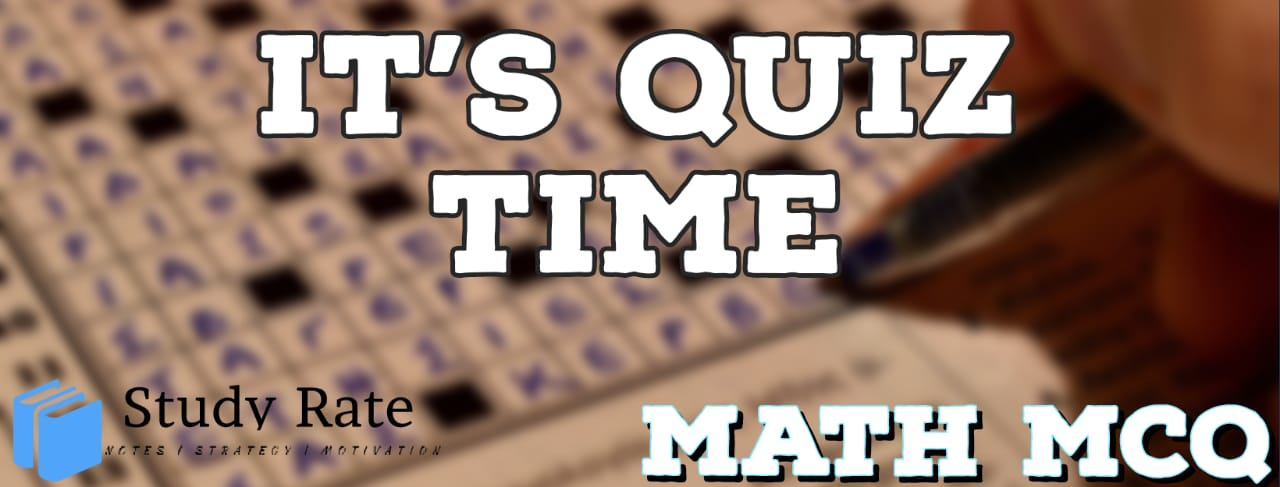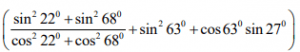## CBSE Class 10 Maths Chapter 8 Introduction to Trigonometry MCQ Quiz

Free PDF Download of CBSE Class 10 Maths Chapter 8 Introduction to Trignometry Multiple Choice Questions with Answers. MCQ Questions for Class 10 Maths with Answers was Prepared Based on Latest Exam Pattern. Students can solve Class 10 Maths Introduction to Trignometry MCQs with Answers to know their preparation level

Introduction to Trigonometry Class 10 Math MCQ’s Online Quiz

1. In a right triangle ABC, the right angle is at B. Which of the following is true about the other two angles A and C?

2. . In a right triangle ABC, the right angle is at B. What is the length of missing side in the figure?3. Which of the following numbers can form sides of a right-angled triangle?

4. Which of the following are Pythagorean triplets?

5. If sin A = 8/17, find the value of secA cosA + cosecA cosA.

6. If 5tanθ=4, then value of (5sinθ -4cosθ)/(5sinθ +4cosθ) is:

7.8. The value ofis:

9. If a pole 6m high casts a shadowlong on the ground, then the sun’s elevation is

10.